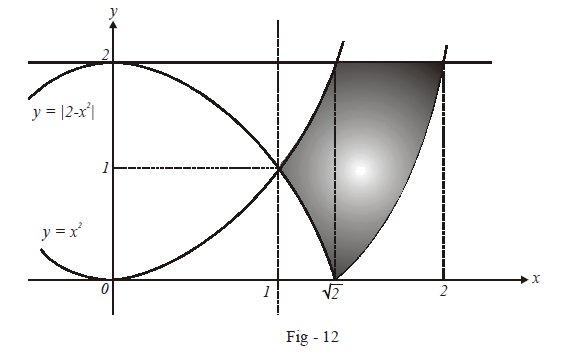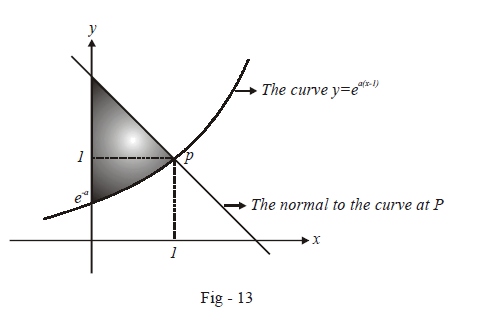# Problems On Areas Set-6

Go back to  'Areas and Volumes'

## Problems on areas examples

Example – 11

Find the area of the region bounded by the curves

${\rm{y = }}{{\rm{x}}^2},{\rm{y = }}\left| {{\rm{2 - }}{{\rm{x}}^2}} \right|{\rm{and\,y = 2}},$

which lies to the right of the line x = 1.

Solution: The required area is sketched belowThe required area can be evaluated as follows:

\begin{align}&{\rm{A = }}\int\limits_1^{\sqrt 2 } {({x^2} - (2 - {x^2}))dx + \int\limits_{\sqrt 2 }^2 {(2 - ({x^2} - 2))dx} } \\&\;\; = \left. {\left( {\frac{{2{x^3}}}{3} - 2x} \right)} \right|_1^{\sqrt 2 } + \left. {\left( {4x - \frac{{{x^3}}}{3}} \right)} \right|_{\sqrt 2 }^2\\& \;\;= \frac{{20 - 12\sqrt 2 }}{3}sq.\rm{units} \qquad{\rm{(\,verify\;the\;calculations)}} \end{align}

Example – 12

A curve $$y = f\left( x \right)$$ passes through the point P (1, 1) . The normal to the curve at P is $$a(y - 1) + (x - 1) = 0$$ . If the slope of the tangent at any point on the curve is proportional to the ordinate of that point, determine the equation of the curve. Hence obtain the area bounded by the y-axis, the curve and the normal to the curve at P.

Solution: The slope of the given normal is obvious from the expression:

\begin{align}&\frac{{y - 1}}{{x - 1}} = - \frac{1}{a}\\&\Rightarrow {m_N} = - \frac{1}{a}\\&\Rightarrow {m_T} = a\\&\Rightarrow {\left. {\left( {\frac{{dy}}{{dx}}} \right)} \right|_p} = a\end{align}

\begin{align}\text{It is given that}\; \frac{{dy}}{{dx}}\propto y \Rightarrow \frac{{dy}}{{dx}} = ky\end{align}

\begin{align}\text {Since}\qquad {\left. {\frac{{dy}}{{dx}}} \right|_{(1,1)}} = a \Rightarrow k = a\end{align}

Thus,

\begin{align}&\frac{{dy}}{{dx}} = ay\\&\Rightarrow \frac{{dy}}{y} = adx\\&\Rightarrow \ln y = ax + {\mathop{\rm lnC}\nolimits} \qquad {\rm{(we\;took\; the\;constant\;of\;integration\;as\;ln\;C\;instead\;}}\\&\qquad \qquad \qquad\qquad\qquad\quad{\rm{of\; C\;so\;that\;the\;final \;expression\;for\;y\;is\;simpler)}}\\&\Rightarrow y = C{e^{ax}}\end{align}

Since this curve passes through (1, 1),

$\begin{array}{l}1 = C{e^{a.1}}\\ \Rightarrow C = {e^{ - a}}\end{array}$

Thus, the equation of the curve is

$y = {e^{a(x - 1)}}$

Let us now proceed to evaluate the bounded area, which is sketched below:The equation of the normal has already been provided:

$y = 1 + \frac{1}{a}(1 - x)$

Thus, the required area is:

\begin{align} A &= \int\limits_0^1 {\left\{ {\left( {1 + \frac{1}{a}\left( {1 - x} \right)} \right) - {e^{a(x - 1)}}} \right\}} dx\\&= \left. {\left( {x + \frac{x}{a} - \frac{{{x^2}}}{{2a}} - \frac{{{e^{a(x - 1)}}}}{a}} \right)} \right|_0^1\\&= \left( {1 + \frac{1}{a} - \frac{1}{{2a}} - \frac{1}{a}} \right) - \left( { - \frac{{{e^{ - a}}}}{a}} \right)\\&= \left( {1 - \frac{1}{{2a}} + \frac{1}{{a{e^a}}}} \right){\rm{sq}}{\rm{. units}}\end{align}